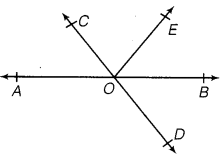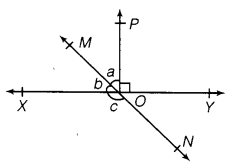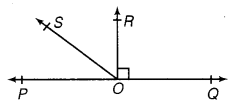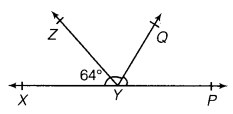# CLASS 9 MATH NCERT SOLUTION FOR CHAPTER – 6 Lines and Angles EX – 6.1

## Lines and Angles

Question 1.
In the figure, lines AB and CD intersect at O. If ∠AOC + ∠BOE = 70° and ∠BOD = 40°, find ∠BOE and reflex ∠COE.Solution:
Here,∠AOC and ∠BOD are vertically opposite angles.
∴  ∠AOC =  ∠BOD
⇒ ∠AOC –  40° [BOD=40° (Given)]…(i)
We have, ∠AOC + ∠BOE = 70° (Given)
40° + ∠BOE =   70° [from eq.(1)]
⇒ ∠BOE =    30°
Also, ∠AOC + ∠COE + ∠BOE – 180° (Linear pair axiom)
⇒  40° + ∠COE + 30° = 180° ∠COE=110°
Now,  ∠COE + reflex ∠COE = 360° (Angles at a point)110° + reflex ∠COE = 360°
⇒ Reflex ∠COE = 250°

Question 2.
In figure lines XY and MN intersect at O. If ∠POY = 90° and a: b = 2: 3 find c.Solution:
We have, ∠POY = 90°
⇒ ∠POY + ∠POX = 180° (Linear pair axiom)
⇒ ∠POX = 90°
⇒ a + b= 90°……….(i)
Also a : b = 2 : 3        (Given)
⇒ Let   a = 2k, b = 3k
Now, from Eq. (i), we get
2k +3k- 90° ⇒ 5k = 90° ⇒ k = 18°
∴ a = 2 x 18° = 36°
and b = 3 x 18°= 54°
Now, ∠MOX + ∠XON = 180° b + c = 180°
⇒ 54° + c = 180°
⇒ c = 126°

Question 3.
In the given figure, ∠PQR = ∠PRQ, then prove that ∠PQS = ∠PRT.Solution:
∠PQS +∠PQR =180°   [linear Pair] …………(i)
∠PRT + ∠PRQ = 180°  [linear Pair] …………(ii)
From eqs. (i) and (ii), we have
∠PQS + ∠PQR =∠PRT + ∠PRQ
⇒  ∠PQS = ∠PRT   [∵ ∠PQR = ∠PRQ (given)]

Question 4.
In the given figure, if x + y= w + z, then prove that AOB is a line.

Solution:

We know that, sum of all the angles around a point is 360°.
∴  x + y + z + w = 360°
⇒ (x + y) + (z + w) = 360°
⇒ (x + y) + (x + y)= 360° [ ∵ x + y = z + w, given]
⇒ 2(x + y) = 360°
⇒ (x + y)=$\cfrac { 360 }{ 2 }$ ⇒ x + y = 180°
So, AOB is a straight line
Hence proved.

Question 5.
In figure, POQ is a line. Ray OR is perpendicular to line PQ. OS is another ray lying between rays OP and OR. Prove that
∠ROS =$\cfrac { 1 }{ 2 }$ (∠QOS – ∠POS)Solution:
We have,  ∠POR = ∠ROQ = 90° (∵ Given that, OR is perpendicular to PQ)
∴  ∠POS + ∠ROS = 90°
⇒  ∠ROS = 90°- ∠POS
On adding ZROS both sides, we get
⇒ 2 ∠ROS = 90°-∠POS + ∠ROS
⇒  2∠ROS = (90°  + ∠ROS) – ∠POS
⇒ 2∠ROS = ∠QOS – ∠POS
(∵ ∠QOS = ∠ROQ + ∠ROS = 90° + ∠ROS)
⇒  ∠ROS =$\cfrac { 1 }{ 2 }$ (∠QOS –∠POS) Hence proved.

Question 6.
It is given that ∠XYZ = 64° and XY is produced to point P. Draw a figure from the given information. If ray YQ bisects ∠ZYP, find ∠XYQ and reflex ∠QYP.

Solution:
Here, YQ bisects ∠ZYP.Hence ∠ZYQ  =$\cfrac { 1 }{ 2 }$ ∠QYP = ∠ZYP  ………..(i)
Given,    ∠XYZ =  64° …………(ii)
∵ ∠XYZ + ∠ZYQ + ∠QYP = 180°  (Linear pair axiom)
⇒ 64° + ∠ZYQ + ∠ZYQ = 180°    [From eq .(i) a and(ii)]
⇒ 2∠ZYQ =  180°-64°
⇒ ∠ZYQ =$\cfrac { 1 }{ 2 }$ x 116° ⇒ ∠ZYQ – 58°
∴  ∠XYQ =  ∠XYZ + ∠ZYQ
⇒ 64° + 58° = 122°
Now, ∠QYZ + reflex ∠QYP = 360°
58° + reflex ∠QYP – 360°    ⇒ reflex ∠QYP = 302

#### CLASS 9 MATH NCERT SOLUTION FOR CHAPTER – 6 LINES AND ANGLES EX – 6.2

Lorem ipsum dolor sit amet, consectetur adipiscing elit. Phasellus cursus rutrum est nec suscipit. Ut et ultrices nisi. Vivamus id nisl ligula. Nulla sed iaculis ipsum.

Company Name